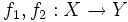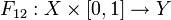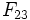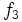# 2-category of topological spaces with continuous maps and homotopies

This article describes a category (in the mathematical sense) whose objects are topological spaces, and whose morphisms are continuous maps. In other words, it gives a category structure to the collection of all topological spaces.
View other category structures on topological spaces

## Definition

The 2-category of topological spaces with continuous maps and homotopies is defined as follows:

Aspect Name Definition/description
objects topological spaces A topological space is a set along with a collection of subsets, called open subsets, that contains the empty subset and the whole space, and is closed under taking arbitrary unions and finite intersections.
morphisms continuous maps between topological spaces A continuous map$f:X \to Y$ of topological spaces is a set map with the property that for every open subset$U$ of$Y$,$f^{-1}(U)$ is an open subset of$X$.
composition law for morphisms compose as set maps Not needed.
2-morphisms homotopies between continuous maps. Given two continuous maps$f_1,f_2:X \to Y$ between topological spaces$X$ and$Y$, a homotopy between$f$ and$g$ is a continuous map$F_{12}:X \times [0,1] \to Y$, where$F_{12}(x,0) = f_1(x)$ and$F_{12}(x,1) = f_2(x)$ for all$x \in X$. Here,$[0,1]$ is the closed unit interval, and$X \times [0,1]$ is given the product topology.
composition law for 2-morphisms do one homotopy after the other The composition law for homotopies is as follows: given a homotopy$F_{12}$ from$f_1$ to$f_2$ and a homotopy$F_{23}$ from$f_2$ to$f_3$, the composite is a homotopy that does$F_{12}$ in the first half of the time, and then does$F_{23}$ in the second half of the time.

## Facts

### Relation with the associated 1-category

If we forget the 2-morphisms, we get the usual category of topological spaces with continuous maps.

### The homotopy category of topological spaces

The 2-category described here is very special in the sense that all its 2-morphisms are invertible. Hence, we can safely take a quotient of the 1-category modulo the equivalence relation induced by the 2-morphisms. This gives rise to the homotopy category of topological spaces, where two continuous maps$f$ and$g$ are identified if they are homotopic.

## Functors to and from the 2-category

### Singular chain complex

Further information: singular chain complex 2-functor

The singular chain complex can be viewed as a 2-functor from this 2-category to the 2-category of chain complexes with chain maps and chain homotopies. This gives a strong form of the homotopy-invariance theorem for singular homology.

### Underlying space of a cellular space

The underlying space functor can be viewed as a 2-functor from the 2-category of cellular spaces with cellular maps and homotopies to this 2-category, which sends each cellular space to its underlying topological space, each cellular map to its underlying continuous map, and each homotopy to the corresponding homotopy.

This in particular gives a 2-functor from the 2-category of CW-complexes with cellular maps and homotopies.